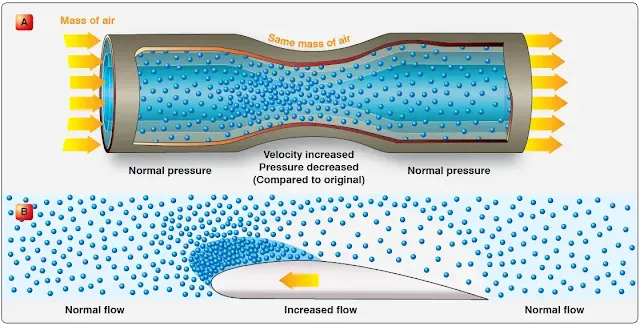# Aerodynamics and the Laws of Physics

The law of conservation of energy states that energy may neither be created nor destroyed.

Motion is the act or process of changing place or position. An object may be in motion with respect to one object and motionless with respect to another. For example, a person sitting quietly in an aircraft flying at 200 knots is at rest or motionless with respect to the aircraft; however, the person and the aircraft are in motion with respect to the air and to the earth.

Air has no force or power, except pressure, unless it is in motion. When it is moving, however, its force becomes apparent. A moving object in motionless air has a force exerted on it as a result of its own motion. It makes no difference in the effect then, whether an object is moving with respect to the air or the air is moving with respect to the object. The flow of air around an object caused by the movement of either the air or the object, or both, is called the relative wind.

## Velocity and Acceleration

The terms “speed” and “velocity” are often used interchangeably, but they do not have the same meaning. Speed is the rate of motion in relation to time, and velocity is the rate of motion in a particular direction in relation to time.

An aircraft starts from New York City and flies 10 hours at an average speed of 260 miles per hour (mph). At the end of this time, the aircraft may be over the Atlantic Ocean, Pacific Ocean, Gulf of Mexico, or, if its flight were in a circular path, it may even be back over New York City. If this same aircraft flew at a velocity of 260 mph in a southwestward direction, it would arrive in Los Angeles in about 10 hours. Only the rate of motion is indicated in the first example and denotes the speed of the aircraft. In the last example, the particular direction is included with the rate of motion, thus, denoting the velocity of the aircraft.

Acceleration is defined as the rate of change of velocity. An aircraft increasing in velocity is an example of positive acceleration, while another aircraft reducing its velocity is an example of negative acceleration, or deceleration.

## Newton’s Laws of Motion

The fundamental laws governing the action of air about a wing are known as Newton’s laws of motion.

Newton’s first law is normally referred to as the law of inertia. It simply means that a body at rest does not move unless force is applied to it. If a body is moving at uniform speed in a straight line, force must be applied to increase or decrease the speed.

According to Newton’s law, since air has mass, it is a body. When an aircraft is on the ground with its engines off, inertia keeps the aircraft at rest. An aircraft is moved from its state of rest by the thrust force created by a propeller, or by the expanding exhaust, or both. When an aircraft is flying at uniform speed in a straight line, inertia tends to keep the aircraft moving. Some external force is required to change the aircraft from its path of flight.

Newton’s second law states that if a body moving with uniform speed is acted upon by an external force, the change of motion is proportional to the amount of the force, and motion takes place in the direction in which the force acts. This law may be stated mathematically as follows:

Force = mass × acceleration (F = ma)

If an aircraft is flying against a headwind, it is slowed down. If the wind is coming from either side of the aircraft’s heading, the aircraft is pushed off course unless the pilot takes corrective action against the wind direction.

Newton’s third law is the law of action and reaction. This law states that for every action (force) there is an equal and opposite reaction (force). This law can be illustrated by the example of firing a gun. The action is the forward movement of the bullet while the reaction is the backward recoil of the gun.

The three laws of motion that have been discussed apply to the theory of flight. In many cases, all three laws may be operating on an aircraft at the same time.

## Bernoulli’s Principle and Subsonic Flow

Bernoulli’s principle states that when a fluid (air) flowing through a tube reaches a constriction, or narrowing, of the tube, the speed of the fluid flowing through that constriction is increased and its pressure is decreased. The cambered (curved) surface of an airfoil (wing) affects the airflow exactly as a constriction in a tube affects airflow. [Figure] Diagram A of Figure illustrates the effect of air passing through a constriction in a tube. In B, air is flowing past a cambered surface, such as an airfoil, and the effect is similar to that of air passing through a restriction.Bernoulli’s Principle

As the air flows over the upper surface of an airfoil, its velocity increases and its pressure decreases; an area of low pressure is formed. There is an area of greater pressure on the lower surface of the airfoil, and this greater pressure tends to move the wing upward. The difference in pressure between the upper and lower surfaces of the wing is called lift. Three-fourths of the total lift of an airfoil is the result of the decrease in pressure over the upper surface. The impact of air on the under surface of an airfoil produces the other one-fourth of the total lift.

RELATED POSTS